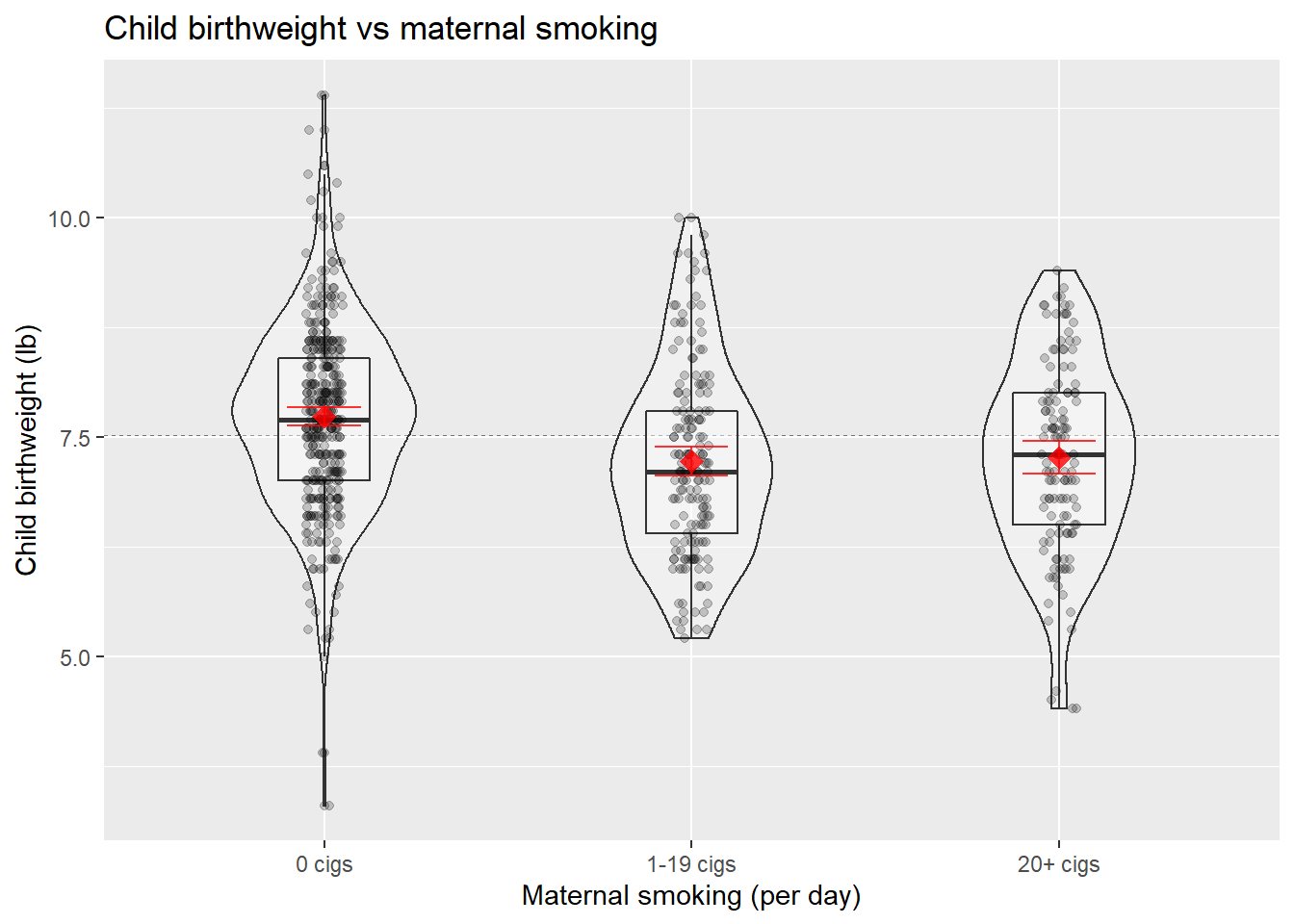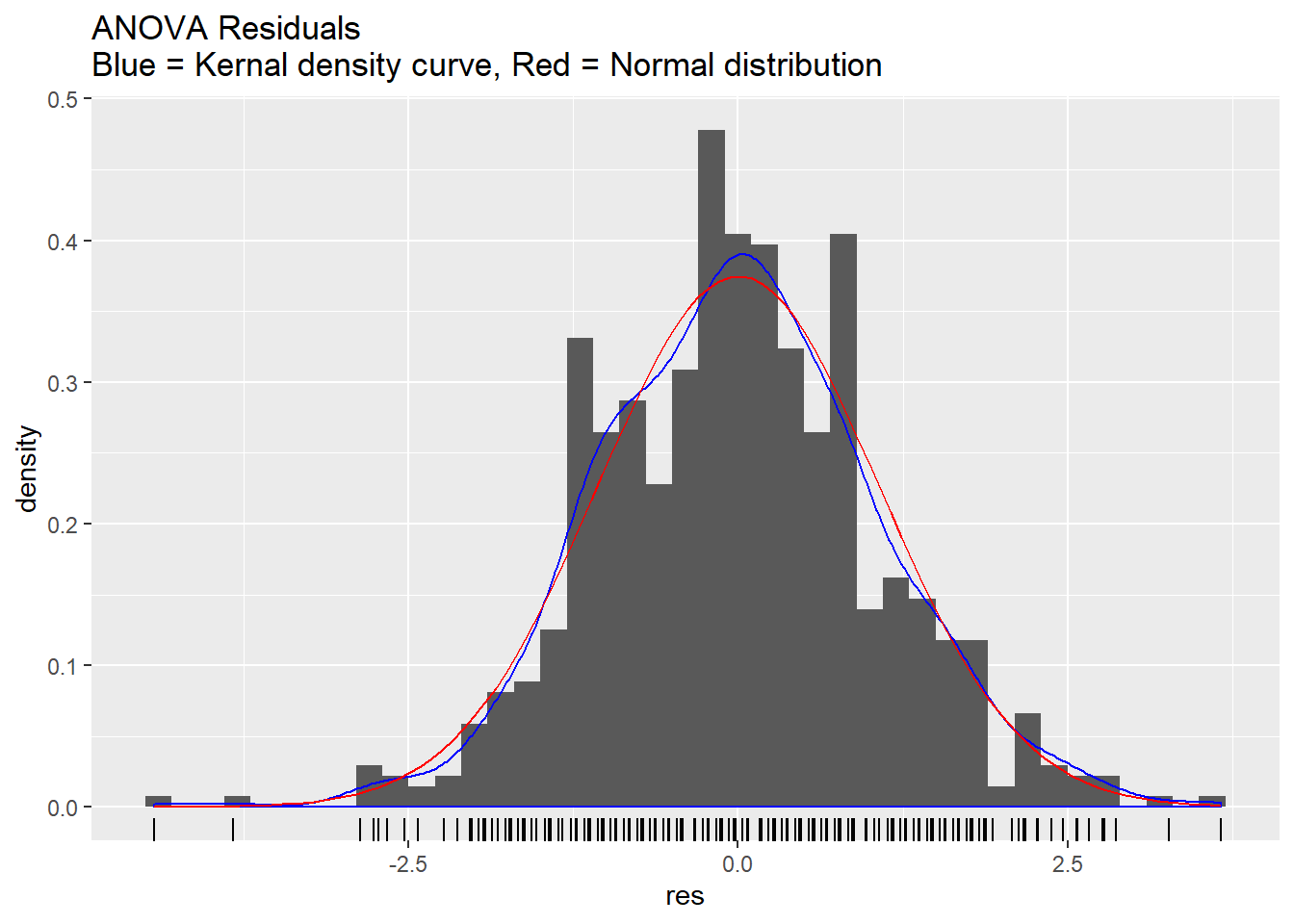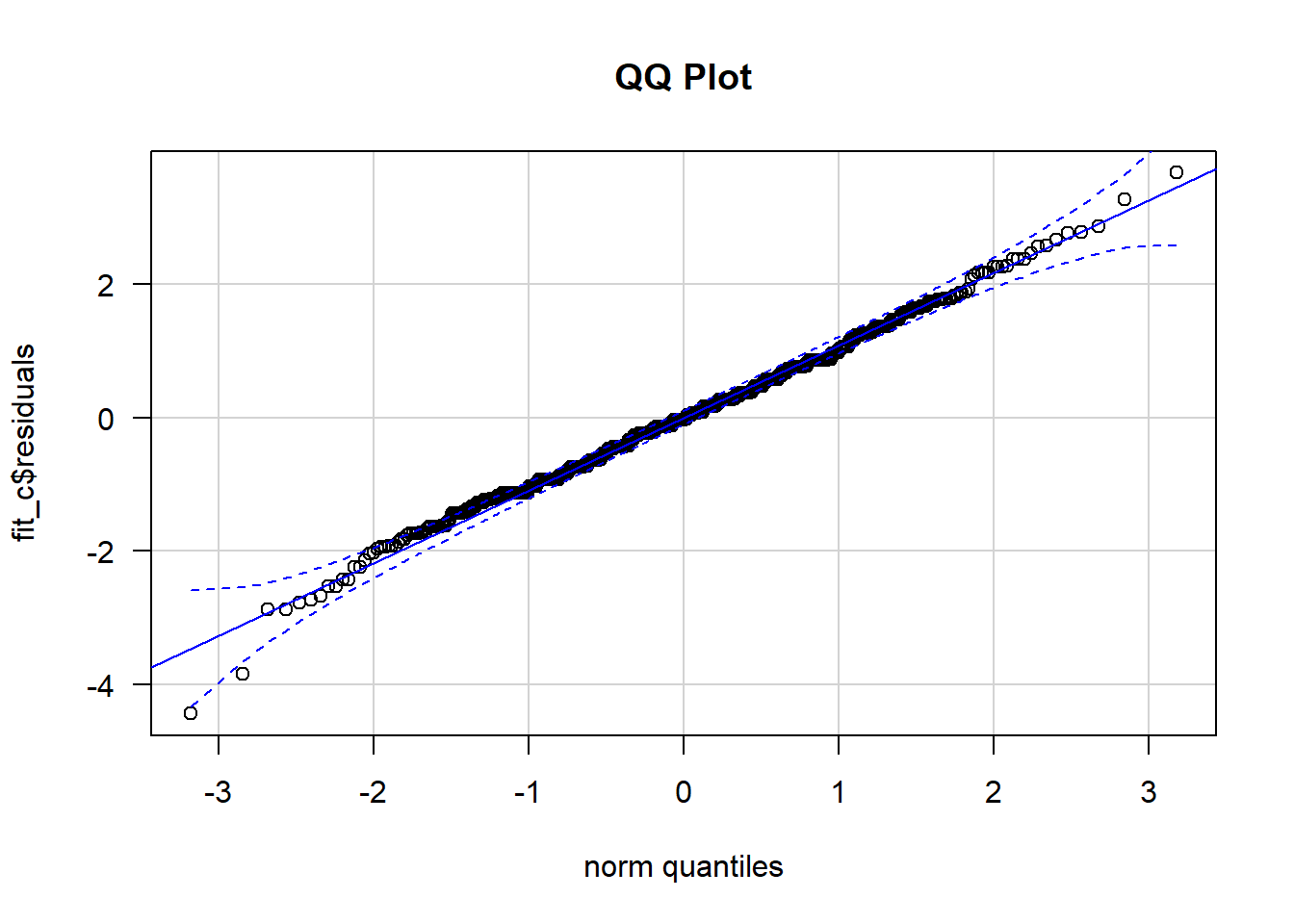Include your answers in this document in the sections below the rubric where I have point values indicated (1 p).

# Rubric

Answer the questions with the data example.

# Example: Child Health and Development Study (CHDS)

We consider data from the birth records of 680 live-born white male infants. The infants were born to mothers who reported for pre-natal care to three clinics of the Kaiser hospitals in northern California. As an initial analysis, we will examine whether maternal smoking has an effect on the birth weights of these children. To answer this question, we define 3 groups based on mother’s smoking history: (1) mother does not currently smoke or never smoked, (2) mother smoked less than one pack of cigarettes a day during pregnancy, and (3) mother smoked at least one pack of cigarettes a day during pregnancy.

Let $$\mu_i$$ = pop mean birth weight (lb) for children in group $$i$$, $$(i=1,2,3)$$. We wish to test $$H_0: \mu_1=\mu_2=\mu_3$$ against $$H_A: \textrm{not } H_0$$.

We read in the data, create a smoke factor variable, and plot the data by smoking group.

library(tidyverse)
## -- Attaching packages ---------------------------------------------------------------------------------------- tidyverse 1.2.1 --
## v ggplot2 3.2.1     v purrr   0.3.2
## v tibble  2.1.3     v dplyr   0.8.3
## v tidyr   1.0.0     v stringr 1.4.0
## v readr   1.3.1     v forcats 0.4.0
## -- Conflicts ------------------------------------------------------------------------------------------- tidyverse_conflicts() --
## x dplyr::lag()    masks stats::lag()
#### Example from the Child Health and Development Study (CHDS)
dat_chds <- read_csv("http://statacumen.com/teach/ADA1/ADA1_notes_05-CHDS.csv")
## Parsed with column specification:
## cols(
##   id = col_character(),
##   c_len = col_double(),
##   c_bwt = col_double(),
##   gest = col_double(),
##   m_age = col_double(),
##   m_smok = col_character(),
##   m_ht = col_double(),
##   m_ppwt = col_character(),
##   p_age = col_double(),
##   p_educ = col_character(),
##   p_smok = col_character(),
##   p_ht = col_double()
## )
dat_chds <-
dat_chds %>%
mutate(
# create a factor variable based on number of cigarettes smoked
smoke = case_when(
# no cigs
m_smok == 0 ~ "0 cigs"
# less than 1 pack (20 cigs = 1 pack)
, (m_smok > 0) & (dat_chds$m_smok < 20) ~ "1-19 cigs" # at least 1 pack (20 cigs = 1 pack) , m_smok >= 20 ~ "20+ cigs" ) , smoke = factor(smoke) ) %>% select( c_bwt , smoke ) summary(dat_chds) ## c_bwt smoke ## Min. : 3.300 0 cigs :381 ## 1st Qu.: 6.800 1-19 cigs:169 ## Median : 7.600 20+ cigs :130 ## Mean : 7.516 ## 3rd Qu.: 8.200 ## Max. :11.400 Plot the data in a way that compares the means. Error bars are 95% confidence intervals of the mean. # Plot the data using ggplot library(ggplot2) p <- ggplot(dat_chds, aes(x = smoke, y = c_bwt)) # plot a reference line for the global mean (assuming no groups) p <- p + geom_hline(yintercept = mean(dat_chds$c_bwt),
colour = "black", linetype = "dashed", size = 0.3, alpha = 0.5)
# boxplot, size=.75 to stand out behind CI
p <- p + geom_violin(width = 0.5, alpha = 0.25)
p <- p + geom_boxplot(width = 0.25, alpha = 0.25)
# points for observed data
p <- p + geom_point(position = position_jitter(w = 0.05, h = 0), alpha = 0.2)
# diamond at mean for each group
p <- p + stat_summary(fun.y = mean, geom = "point", shape = 18, size = 4,
colour = "red", alpha = 0.8)
# confidence limits based on normal distribution
p <- p + stat_summary(fun.data = "mean_cl_normal", geom = "errorbar",
width = .2, colour = "red", alpha = 0.8)
p <- p + labs(x     = "Maternal smoking (per day)"
, y     = "Child birthweight (lb)"
, title = "Child birthweight vs maternal smoking"
)
print(p)Hypothesis test

1. Set up the null and alternative hypotheses in words and notation.

• In words: The population mean birthweight is different between smoking groups.’’
• In notation: $$H_0: \mu_1=\mu_2=\mu_3$$ versus $$H_A: \textrm{not } H_0$$ (at least one pair of means differ).
2. Let the significance level of the test be $$\alpha=0.05$$.

3. Compute the test statistic.

fit_c <- aov(c_bwt ~ smoke, data = dat_chds)
summary(fit_c)
##              Df Sum Sq Mean Sq F value   Pr(>F)
## smoke         2   40.7  20.351    17.9 2.65e-08 ***
## Residuals   677  769.5   1.137
## ---
## Signif. codes:  0 '***' 0.001 '**' 0.01 '*' 0.05 '.' 0.1 ' ' 1

The $$F$$-statistic for the ANOVA is $$F = 17.9$$.

1. Compute the $$p$$-value from the test statistic.

The p-value for testing the null hypothesis is $$p = 2.65\times 10^{-8}$$.

1. (2 p) State the conclusion in terms of the problem.

2. Check assumptions of the test.

1. Residuals are normal
2. Populations have equal variances.
• Check whether residuals are normal.

• Plot the residuals and assess whether they appear normal.

# Plot the data using ggplot
df.res <- data.frame(res = fit_c$residuals) library(ggplot2) p <- ggplot(df.res, aes(x = res)) p <- p + geom_histogram(aes(y = ..density..), binwidth = 0.2) p <- p + geom_density(colour = "blue") p <- p + geom_rug() p <- p + stat_function(fun = dnorm, colour = "red", args = list(mean = mean(df.res$res), sd = sd(df.res$res))) p <- p + labs(title = "ANOVA Residuals\nBlue = Kernal density curve, Red = Normal distribution") print(p)(1 p) Describe the plot of residuals as it relates to model assumptions. • Plot the residuals versus the normal quantiles. If the residuals are normal, then the will fall on the center line and very few will be outside the error bands. # QQ plot par(mfrow=c(1,1)) library(car) ## Loading required package: carData ## ## Attaching package: 'car' ## The following object is masked from 'package:dplyr': ## ## recode ## The following object is masked from 'package:purrr': ## ## some qqPlot(fit_c$residuals, las = 1, id = list(n = 0, cex = 1), lwd = 1, main="QQ Plot")(1 p) Describe the plot of residuals as it relates to model assumptions.

• A formal test of normality on the residuals tests the hypothesis $$H_0:$$ The distribution is Normal vs $$H_1:$$ The distribution is not Normal. We can test the distribution of the residuals.

Three tests for normality are reported below. I tend to like the Anderson-Darling test. Different tests have different properties, and tests that are sensitive to differences from normality in the tails of the distribution are typically more important for us (since deviations in the tails are more influential than deviations in the center).

shapiro.test(fit_c$residuals) ## ## Shapiro-Wilk normality test ## ## data: fit_c$residuals
## W = 0.99553, p-value = 0.04758
library(nortest)
ad.test(fit_c$residuals) ## ## Anderson-Darling normality test ## ## data: fit_c$residuals
## A = 0.62184, p-value = 0.1051
cvm.test(fit_c$residuals) ## ## Cramer-von Mises normality test ## ## data: fit_c$residuals
## W = 0.091963, p-value = 0.1449

(1 p) Interpret the conclusion of the Anderson-Darling test.

• Check whether populations have equal variances.

• Look at the numerical summaries below.

# calculate summaries
dat_chds_summary <-
dat_chds %>%
group_by(smoke) %>%
summarise(
m = mean(c_bwt)
, s = sd(c_bwt)
, n = n()
) %>%
ungroup()

dat_chds_summary
## # A tibble: 3 x 4
##   smoke         m     s     n
##   <fct>     <dbl> <dbl> <int>
## 1 0 cigs     7.73  1.05   381
## 2 1-19 cigs  7.22  1.08   169
## 3 20+ cigs   7.27  1.09   130

(1 p) Interpret the standard deviations above. You may also discuss the plots of the data.

• Formal tests for equal variances. We can test whether the variances are equal between our three groups. This is similar to the ANOVA hypothesis, but instead of testing means we’re tesing variances. $$H_0: \sigma^2_1=\sigma^2_2=\sigma^2_3$$ versus $$H_A: \textrm{not } H_0$$ (at least one pair of variances differ).
## Test equal variance
# assumes populations are normal
bartlett.test(c_bwt ~ smoke, data = dat_chds)
##
##  Bartlett test of homogeneity of variances
##
## data:  c_bwt by smoke
## Bartlett's K-squared = 0.3055, df = 2, p-value = 0.8583
# does not assume normality, requires car package
library(car)
leveneTest(c_bwt ~ smoke, data = dat_chds)
## Levene's Test for Homogeneity of Variance (center = median)
##        Df F value Pr(>F)
## group   2  0.7591 0.4685
##       677
# nonparametric test
fligner.test(c_bwt ~ smoke, data = dat_chds)
##
##  Fligner-Killeen test of homogeneity of variances
##
## data:  c_bwt by smoke
## Fligner-Killeen:med chi-squared = 2.0927, df = 2, p-value = 0.3512

(1 p) Interpret the result of the appropriate test. If normality was reasonable then use Bartlett, otherwise use Levene.

1. If the ANOVA null hypothesis was rejected, then perform follow-up Post Hoc pairwise comparison tests to determine which pairs of means are different.

There are several multiple comparison methods described in the notes. Let’s use Tukey’s Honest Significant Difference (HSD) here to test which pairs of populations differ.

## CHDS
# Tukey 95% Individual p-values
TukeyHSD(fit_c)
##   Tukey multiple comparisons of means
##     95% family-wise confidence level
##
## Fit: aov(formula = c_bwt ~ smoke, data = dat_chds)
##
## \$smoke
##                           diff        lwr        upr     p adj
## 1-19 cigs-0 cigs   -0.51150662 -0.7429495 -0.2800637 0.0000008
## 20+ cigs-0 cigs    -0.46665455 -0.7210121 -0.2122970 0.0000558
## 20+ cigs-1-19 cigs  0.04485207 -0.2472865  0.3369907 0.9308357

(2 p) Interpret the comparisons (which pairs differ).

(1 p) Summarize results by ordering the means and grouping pairs that do not differ (see notes for examples).

Replace this example with your results.

Example:  Groups A and C differ, but B is not different from either.
(These groups are ordered by mean, so A has the lowest mean and C has the highest.)

Group A   Group B   Group C
-----------------
-----------------